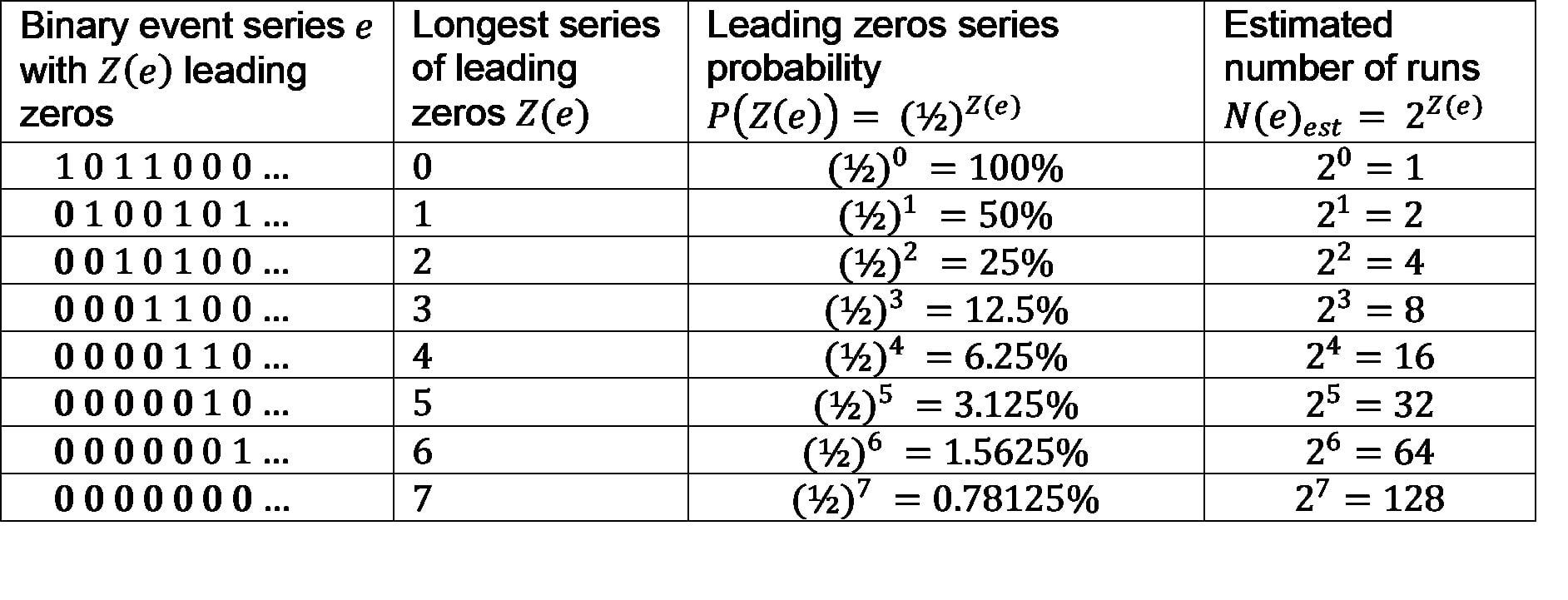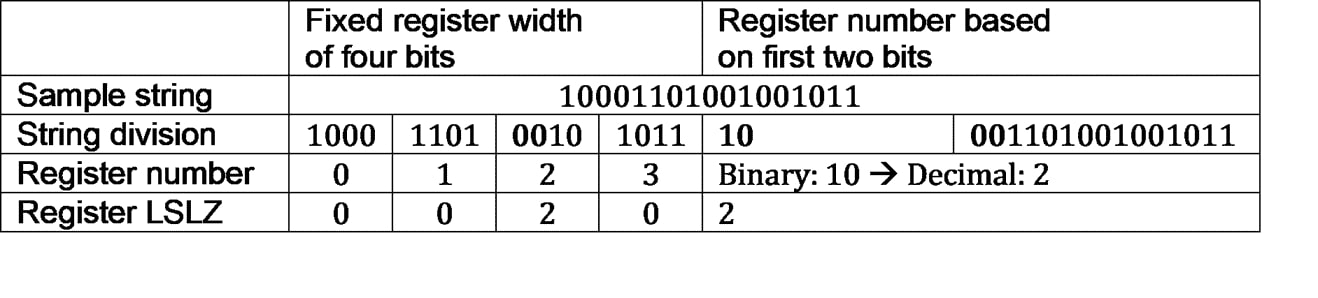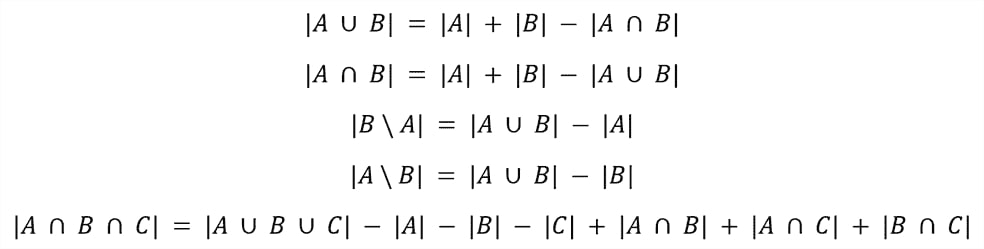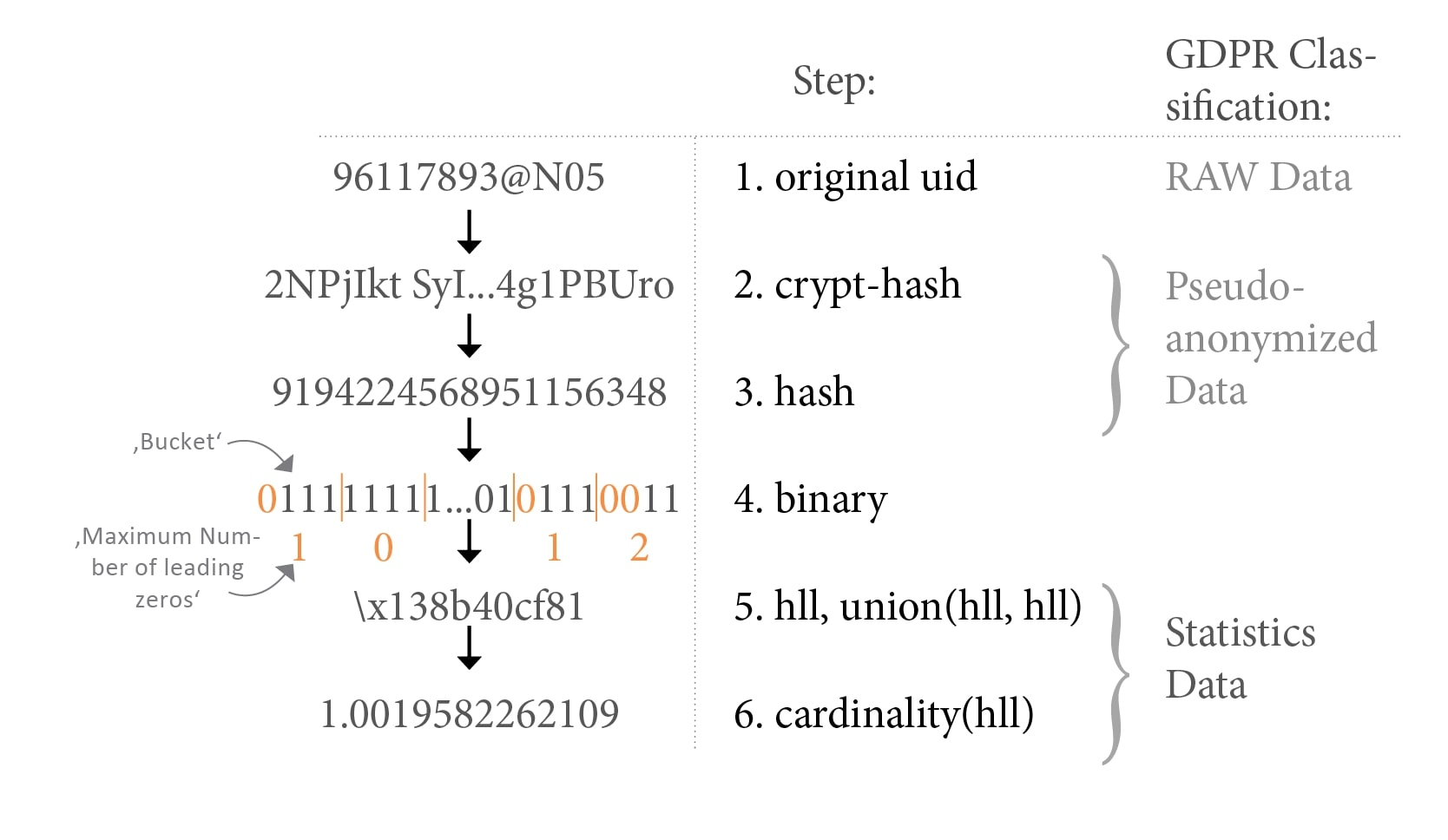Have you ever wondered how HyperLogLog works? Or have you never heard of it at all? In this post I explain the wonderful algorithm of Flajolet et al. from scratch and in a very simple manner.

## Motivation

Currently I’m writing my Master’s thesis about a privacy-aware Social Media dashboard using HyperLogLog as data structure. I remember that most scientific papers had quite a steep learning curve and that it was hard to find a very basic explanation online in the very beginning. There were to many lose ends to combine (coin tosses, probabilities, registers, hashes, binary numbers, logical operators…) and the gap from a simple Laplace coin experiment to a real life application was too far to imagine.

## Watch these videos first

Luckily there are two really helpful videos ,  I would highly recommend watching if you have no clue about HLL, even before reading this post.

## HyperLogLog for everyone

I wanted to create a very simple, logical and incrementing explanation for everyone.

As this explanation originates from a chapter of my Master’s thesis it involves scientific quotes and uses examples in the location-based Social Media (LBSM) context.

Let’s start!

# The Count-Distinct Problem

The count-distinct problem deals with how cardinalities can be calculated as efficiently and accurately as possible, that is how distinct elements can be identified from a set.

Too complicated? Imagine an excel-sheet where every row represents one Instagram post in your city. There is a column for the text, the date, the location, a post ID and most importantly: a user ID.

If your task was to find how many different people (=distinct) posted something, how would you do this?

You would simply use the user-ID column, look at every single user-ID and add +1 to an imaginary counter if the user-ID wasn’t seen before. For excel there is a useful function for this, which is called “Remove Duplicates”.

User IDs of a hypothetical post list… after removing duplicates
U-123U-123
U-123U-984
U-984U-284
U-123
U-123
U-984
U-284
U-123

Here you see that our initial 8 posts were posted by only 3 users. So, the cardinality would be 3. Easy right?

# Social Media Context

In the context of location-based Social Media, this is an elementary problem that can already provide important information on visit frequencies, utilization rates, or potential analyses.

A simple example would be how many users were at a certain place in a certain period of time or how many posts with certain terms were posted in a certain period of time to get exactly this information.

With original data, this could be solved by simple SQL filters, for example, by filtering the posts by time period, location and any terms they contain. This results in a set of posts, within which a unique identifier (UID), such as a user ID (available on Facebook, Instagram, Twitter, etc.), but also an email address, telephone number, etc., can be used to determine how many unique elements are in this set using a classic count-distinct algorithm. If, for example, one is interested in how many different users regularly post in one place, each user may consequently only be counted once, regardless of how much each user posts. This approach is problematic for two reasons.

# The Problems with Original Data

On the one hand, the computational effort and storage requirements increase quasi proportionally to the amount of posts, which, in view of the Big Data character of LBSM data, would lead to cost-intensive computations of powerful central processing units (CPUs) and constantly expanding storage capacities. For large corporations like Google and Facebook with near-infinite financial possibilities, this likely isn’t a huge problem. However, if such a dashboard is to be hosted by a municipality or even by individuals, this would already be an exclusion criterion depending on the means.

On the other hand, different elements in a subset can only be identified if UIDs are permanently stored and extended with all metadata like timestamp (data, time), location (coordinate, location ID o.s.) and so on. This procedure is thus ruled out for a potentially public dashboard.

And this is exactly where HLL comes in, as it is not only able to reduce storage and computational requirements to a minimum, but also to guarantee privacy through its probabilistic structure when used correctly, as no individual can be re-identified from the HLL format if the set is big enough. Furthermore, it does not need to store the original data at any time, but allows to process it directly in-memory.

The HLL method was developed by a group of French researchers around the mathematician Philippe Flajolet (cf. Flajolet & Martin 1985; cf. Flajolet et al. 2007). It provides a solution to the count-distinct problem with a special focus on low memory and computational resources by transforming original data into probabilistic data.

The core idea of HLL can be illustrated by a Laplace coin toss experiment. Given a coin with a 1 (heads) on one side and a 0 (tails) on the other, where both sides are equally likely to occur (50%).

The probability in an experiment where the coin is tossed five times to get the first four times the zero is improbable. More precisely, this probability is

1/2 * 1/2 * 1/2 * 1/2 = (1/2)^4 = 1∕16 = 0.0625 = 6.25%

However, if this experiment is performed often enough, the probability increases proportionally, since each run of five tosses has the same probability.

10 1 0 1 11
21 1 1 0 10
30 0 1 0 02
41 0 1 0 10
50 0 0 1 03
140 0 0 0 14

This table shows a hypothetical experiment with 14 runs, each of which was tossed five times. The leading zeros (LZ) are bold. On the 14th time, the first four tosses are a 0, which is the longest series of leading zeros (LSLZ).

The idea of HLL is now exactly the opposite, to derive from the LSLZ how many runs there were. Assume that the LSLZ is five. It can be derived that the probability for this is

(1/2)^5 = 1/32 = 0.03125 = 3.125%

Thus, on average, five LZs will occur in only one out of 32 cases. So one would estimate that there were about 32 runs in total.In practice, however, the binary strings do not represent random coin tosses, but actual information such as user IDs.

Assuming that these hypothetical user IDs are randomly assigned, they only need to be converted from UTF-8 to binary notation. There are very simple methods to convert them, like firing a simple request in duckduckgo with the addition “to binary”.

If you prefer a solution in plain JavaScript, open a console in your browser with F12, paste the code and see yourself.

 ``````1 2 3 `````` ``````"U123".split("").reduce(function (a, b) {return a + b.charCodeAt(0).toString(2)}, "") // Result "1010101110001110010110011" ``````

The user ID "U123" leads to "1010101110001110010110011" written in binary.

# The Most Basic HLL Application

Now a first strongly simplified version of HyperLogLog could already be applied to a simple example. If one has 10,000 user IDs which were all assigned randomly, one would convert these all into their binary format. In the second step the LSLZ would be identified, which can be done either sequentially or in parallel.

For this example it is sufficient to store only the LSLZ for the whole HLL set which requires correspondingly little memory.

Now, additional elements can be added very easily by simply identifying the LSLZ for each additional string to be added. If this is larger than for the entire HLL set, the LSLZ would be adjusted accordingly. If it is smaller, the LSLZ for the HLL set remains the same.

## HLL Unions

Analogously, entire HLL sets can be combined with each other if the question is, for example, how many unique users were at two locations.

To read out the HLL set, based on the LSLZ it is estimated how many elements have been read into this set.

## No Removal

Individual elements cannot be removed retrospectively because there is no unique assignment due to the fact that only the first digits are considered. Accordingly, it is also not possible to check whether a specific element e was read into the set1.

# Going Further – HyperLogLog Theory

The method described so far is a highly simplified version of HLL, which has a high error rate. Flajolet et al. (cf. 2007) extend it to obtain more accurate estimates. By dividing the binary string into so-called registers (ibid.: 129), i.e. sections, an accuracy of the estimate of about 2% can be obtained (ibid.: 127).

For example, a binary string can be divided into previously defined registers of n bits each. The LSLZ is then only counted for the respective register.

Alternatively, the first N bits can be used as random binary register number and the rest for the register LSLZ. Depending on how many bits are to serve as register number, one gets a certain number of registers in binary logic. For example, if the first two bits are selected, four different registers are used: 00, 01, 10 and 11, which in decimal notation correspond to 0, 1, 2 and 3.For cardinality estimation, Flajolet et al. (ibid.) form the harmonic mean of the probability estimates from all registers (ibid.:129).

# HLL operators

Main HLL operators are so-called unions (Dunkel, Löchner & Burghardt 2020: 8) which are lossless (Garcia-Recuero 2021: 2) and intersections (ibid.: 12) producing rather high error rates (Garcia-Recuero 2021: 2, ) which will be taken up later.

Unions and intersections follow the inclusion-exclusion-principle (ibid.: 4; Ertl 2017: 2, 34).

For the elements A, B and respectively C the following formula apply:(Andreescu & Feng 2004: 117ff; Ertl 2017: 34; Dunkel, Löchner & Burghardt 2020: 12).

With unions one can answer rather complex questions. Assuming multiple different HLL-Sets, one for each urban green space in a city, the question of how many distinct people in the city post about urban green spaces could simply be solved by a union of all HLL-Sets.

# The need for hashing

Imagine a non-random distribution of UIDs which is almost always the case in real-life SM. Users might have certain preferences in user names, numbers etc. but also a company could choose to add a certain prefix to an UID. If i.e. the UIDs of a company always start with the prefix “User-” and this would hypothetically translate to eight LZ right in the beginning of any bit string, this would interfere with the HLL assumption of an even distribution.

Hash-function can help with this. A hash function is a function that maps a string with an arbitrary number of characters to another string with a fixed number of characters without collision (Damgård 1989: 416). A user-ID "User-123456789" could thus be mapped to a string of e.g. six characters e.g. "x9jQwR". This in turn can be represented binary, i.e. only with ones and zeros. In addition, there are certain specifics, such as the greatest possible difference between the hashes of similar input IDs. For example, the hash of "User-123456789" must be very different from the hash of "User-123456788" and should not be "x9jQwT".

For a real-life implementation a uniform hash function is vital. Only if the hash function is capable of distributing bits “over all m registers according to a multinomial distribution with equal probabilities” (Ertl 2017: 2) it is suited for usage in combination with HLL.

Additionally, Dunkel, Löchner & Burghardt use cryptographic hashing as proposed by Desfontaines, Lochbihler, & Basin (2019: 27f) as an additional step to make HLL-Sets more secure and private (2020:7). The original UID can firstly be hashed cryptographically. Afterwards it is hashed for an even distribution, converted to binary and read into an HLL-Set.This figure is taken from Dunkel, Löchner & Burghardt (2020: 7) and sums up everything learnt so far. Also check out their slides.

If you want to find out more about a practical implementation of HLL in the context of Volunteered Geographic Information, go ahead and read their paper.

I hope this explanation was useful! If you would like to continue reading on the topic, see my other blog posts on how you can use HLL in combination with leaflet hexbins or how you can make a Postgres HLL backend talk to a HLL frontend !

Stay tuned for my Master’s thesis I will publish here within a few months!

P.S.: As I wrote most of these chapters in Word, I converted it with pandoc which worked very well for simple text. Unfortunately for formulas and tables I needed to correct almost everything. But with the help of table to markdown this is done really quickly (if you have no combined cells like I did ;) )

Andreescu, T., & Feng, Z. (2004). Inclusion-exclusion principle. In A path to combinatorics for undergraduates (pp. 117-141). Birkhäuser, Boston, MA.

Ertl, O. (2017). New cardinality estimation algorithms for hyperloglog sketches. arXiv preprint arXiv:1702.01284.

Damgård, I. B. (1989). A design principle for hash functions. In Conference on the Theory and Application of Cryptology (pp. 416-427). Springer, New York, NY.

Desfontaines, D., Lochbihler, A. & Basin, D. (2019). Cardinality Estimators do not Preserve Privacy. Proceedings on Privacy Enhancing Technologies,2019(2) 26-46.

Dunkel, A., Löchner, M., & Burghardt, D. (2020). Privacy-Aware Visualization of Volunteered Geographic Information (VGI) to Analyze Spatial Activity: A Benchmark Implementation. ISPRS International Journal of Geo-Information, 9(10), 607.

Flajolet, P., Fusy, É., Gandouet, O., & Meunier, F. (2007). Hyperloglog: the analysis of a near-optimal cardinality estimation algorithm. In Discrete Mathematics and Theoretical Computer Science (pp. 137-156). Discrete Mathematics and Theoretical Computer Science.

Flajolet, P. & Martin, G. N. (1985). Probabilistic counting algorithms for data base applications. Journal of computer and system sciences, 31(2), 182-209.

Garcia-Recuero, A. (2021). Approximate Privacy-Preserving Neighbourhood Estimations. arXiv preprint arXiv:2102.12610.

1. For exactly this purpose, to check whether an element e occurs in a subset m, the Bloom Filter was developed, which is based on similar principles. It is not suitable for a privacy-aware dashboard, since user IDs should not be traceable. ↩︎